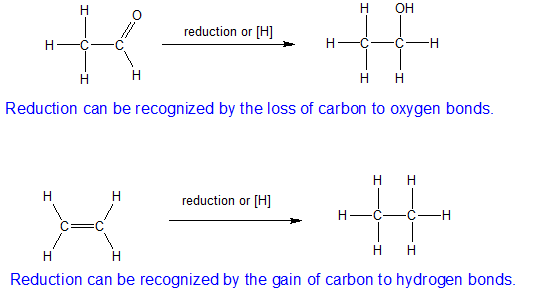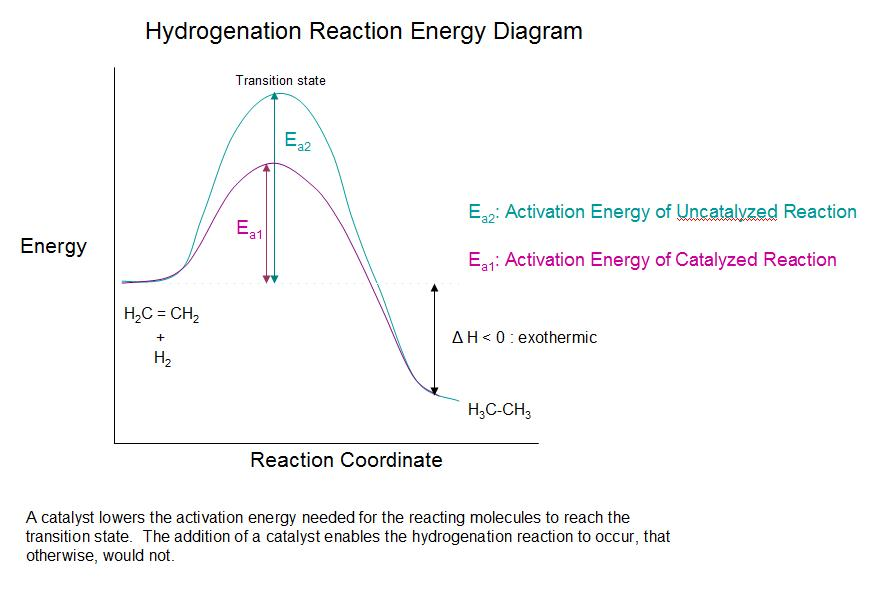# 9.11: Reduction of Alkenes - Catalytic Hydrogenation

Learning Objective
• recognize organic oxidation and reduction reactions
• predict the products/specify the reagents for hydrogenation (reduction) of alkenes

### Introduction

Addition of hydrogen to a carbon-carbon double bond to form an alkane is a reduction reaction that is also called catalytic hydrogenation. Hydrogenation of a double bond is a thermodynamically favorable reaction because it forms a more stable (lower energy) product. In other words, the energy of the product is lower than the energy of the reactant; thus it is exothermic (heat is released). The heat released is called the heat of hydrogenation, which is an indicator of a molecule’s stability. Regioselectivity is not an issue because the same group (a hydrogen atom) is bonded to each of the vinyl carbons. The simplest source of two hydrogen atoms is molecular hydrogen (H2), but mixing alkenes with hydrogen does not result in any discernible reaction. Although the overall hydrogenation reaction is exothermic, a high activation energy prevents it from taking place under normal conditions. This restriction may be circumvented by the use of a catalyst, as shown in the reactions below.## The O-chem View of Oxidation and Reduction

For inorganic chemistry, the flow of electrons is easily counted with the change in oxidation numbers of the metals and non-metals. The expressions "LEO says GER" for "Loss of Electrons is Oxidation and Gain of Electrons is Reduction" or "OIL RIG" for "Oxidation Is Loss and Reduction Is Gain" can be useful guides to recognizing oxidation and reduction reactions for inorganic chemistry. However for organic chemistry, most of the reactants and products are neutral so the electron flow is more difficult to track. For organic compounds, oxidation and reduction reactions can be recognized at least three different ways.

1) Oxidation is an increase in the number of carbon to oxygen bonds or a decrease in the number of carbon to hydrogen bonds.2) Reduction is the opposite of oxidation so it is a decrease in the number of carbon to oxygen bonds or an increase in the number of carbon to hydrogen bonds.3) For reactions that do not involve a change in the bonding of carbon with oxygen and hydrogen, then we need to look at the differences in electronegativity. The shared electrons are assigned to the more electronegative element to determine the oxidation numbers.## The Catalyst

The reaction between hydrogen (H2) gas and an alkene (a carbon-carbon double bond) requires an active metal catalyst. A catalyst increases the reaction rate by lowering the activation energy of the reaction. Although the catalyst is not consumed in the reaction, it is required to accelerate the reaction sufficiently to be observed in a reasonable amount of time. Catalysts commonly used in alkene hydrogenation are: platinum, palladium, and nickel. The metal catalyst acts as a surface on which the reaction takes place. This increases the rate by putting the reactants in close proximity to each other, facilitating interactions between them. With this catalyst present, the sigma bond of H2 breaks, and the two hydrogen atoms instead bind to the metal (see #2 in the figure below). The $$\pi$$ bond of the alkene weakens as it also interacts with the metal as shown in step #3 of the diagram below.Since both the reactants are bound to the metal catalyst, the hydrogen atoms can easily add, one at a time, to the previously double-bonded carbons as shown in steps #4 and #5 above. The position of both of the reactants bound to the catalyst makes it so the hydrogen atoms are only exposed to one side of the alkene. This explains why the hydrogen atoms add to same side of the molecule, called syn-addition.

## Alkene Stability and Catalytic Hydrogenation

As shown in the reaction energy diagram below, the hydrogenation of alkenes is exothermic, and heat is released corresponding to the ΔE (colored green).This heat of reaction can be used to evaluate the thermodynamic stability of alkenes having different numbers of alkyl substituents on the double bond. For example, the following table lists the heats of hydrogenation for three C5H10 alkenes which give the same alkane product (2-methylbutane). Since a large heat of reaction indicates a high energy reactant, these heats are inversely proportional to the stabilities of the alkene isomers. To a rough approximation, we see that each alkyl substituent on a double bond stabilizes this functional group by a bit more than 1 kcal/mole.

 Alkene Isomer Heat of Reaction ( ΔHº ) (CH3)2CHCH=CH2 3-methyl-1-butene CH2=C(CH3)CH2CH3 2-methyl-1-butene (CH3)2C=CHCH3 2-methyl-2-butene –30.3 kcal/mole –28.5 kcal/mole –26.9 kcal/mole

## Stereochemistry of Catalytic Hydrogenation

From the mechanism shown below, we expect the addition of hydrogen to occur with syn-stereoselectivity since both reactants approach the same side of the catalyst's surface.For example, 1,2-dimethylcyclopentene is reduced to 1,2-dimethylcyclopentane during catalytic hydrogenation.Exercises
1. Use the catalytic hydrogenation of ethene with platinum oxide to answer the following questions.

1. 0.500 mol of ethene reacts with _______ mol of hydrogen.
2. Ethene is being _______; while _______ is being oxidized.
3. The oxidation number of carbon in ethene is _______; in ethane it is _______.
2. When 1.000 g of a certain triglyceride (fat) is treated with hydrogen gas in the presence of Adams’ catalyst, it is found that the volume of hydrogen gas consumed at 99.8 kPa and 25.0°C is 162 mL. A separate experiment indicates that the molar mass of the fat is 914 g mol−1. How many carbon-carbon double bonds does the compound contain?

3. Bromobutene reacts with hydrogen gas in the presence of a platinum catalyst. What is the name of the product?
4. Cyclohexene reacts with hydrogen gas in the presence of a palladium catalyst. What is the name of the product?
5. What is the stereochemistry of an alkene hydrogenation reaction?
6. When looking at their heats of hydrogenation, is the cis or the trans isomer generally more stable?
7. 2-chloro-4-ethyl-3methylcyclohexene reacts with hydrogen gas in the presence of a platinum catalyst. What is the name of the product?
8.9. Predict the products if the following alkenes were reacted with catalytic hydrogen.1. a. 0.500 mole of hydrogen gas

b. Ethene is being reduced; while hydrogen is being oxidized.

c. The oxidation number of carbon in ethene is −2; in ethane it is −3.

2. Amount of hydrogen consumed $\begin{array}{l}=n\text{\hspace{0.17em}}\text{mol}\\ \text{=}\frac{PV}{RT}\\ =\frac{99.8\text{\hspace{0.17em}}\text{kPa}×0.162\text{\hspace{0.17em}}\text{L}}{8.31\text{\hspace{0.17em}}\text{kPa}\cdot {\text{mol}}^{-1}\cdot {\text{K}}^{-1}×298\text{\hspace{0.17em}}\text{K}}\\ =6.53×{10}^{-3}\text{\hspace{0.17em}}\text{mol}\text{\hspace{0.17em}}{\text{H}}_{2}\end{array}$

Amount of fat used $\begin{array}{l}=\frac{\left(1.000\text{\hspace{0.17em}}\text{g}\right)×\left(1\text{\hspace{0.17em}}\text{mol}\right)}{\left(914\text{\hspace{0.17em}}\text{g}\right)}\\ =1.09×{10}^{-3}\text{\hspace{0.17em}}\text{mol}\text{\hspace{0.17em}}\text{fat}\end{array}$

Ratio of moles of hydrogen consumed to moles of fat $\begin{array}{l}=6.53×{10}^{-3}:1.09×{10}^{-3}\\ =6:1\end{array}$

Thus, the fat contains six carbon-carbon double bonds per molecule.

3. Bromobutane

4. Cyclohexane

8.